Select Page

# Turing Machine For 0^N1^N

Design a Turing Machine which recognizes the language L = 0N1N where N>0.

Given language (L = 0N1N) will generate equal number of 0’s and 1’s. So, consider a input-tap which hold L=”000111” as given below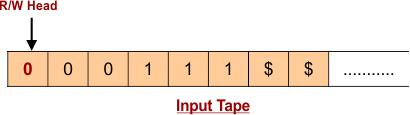## Algorithm For Language (L = 0N1N)

Algorithm for above language in Turing Machine to Accept is given below

• Step 01: Change “0” to “X”
• Step 02: Move Right to First “1”.If no “1” found then simply reject the language
• Step 03: Change “1” to “Y”
• Step 04: Move Left to Leftmost “0”
• Step 05: Repeat Step 01 to 04 until no more “0” and “1” remain in the input tape.

Let construct Turing Machine for a string “000111” of given Language.

According to Step 01, For the first Input “0”

• Transition goes from initial state (q0) to state (q1).
• The first symbol “0” of input tape is updated to “X”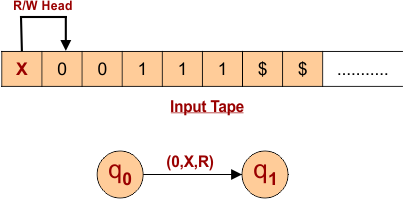According to Step 2 and 3 ,R/W Head Move to Right To update  first “1” in right side of input Tape

• Transition goes from state (q1) to state (q2).
• The symbol “1” of input tape is updated to “Y”
• R/W Head Move one Position Left to update Leftmost “0” in the next.

At q1, transition will remain in same state for input “0” as given below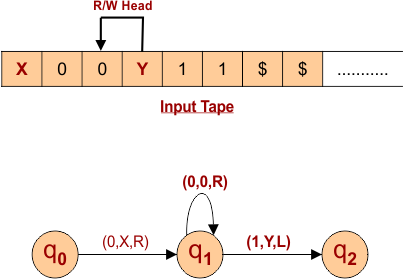According To Step 4 and 01, Move Left to Leftmost “0” and update it to “X”, As

• Transition goes from state (q2) to state (q1) for input “X”
• The Leftmost “0” of input tape is updated to “X”
• After Update Leftmost “0” to “X”, R/W head move to Right for upcoming “1” updating.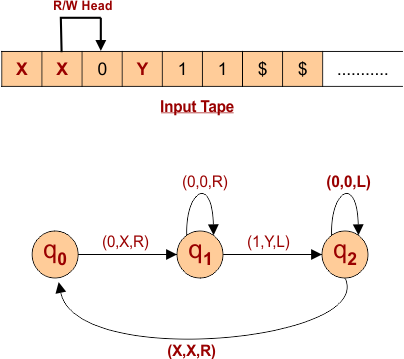Again, According to Step 2 and 3, R/W Head Move to Right To update  first “1” in right side of input tape.

Input tape and Turing machine diagram explain the detail in below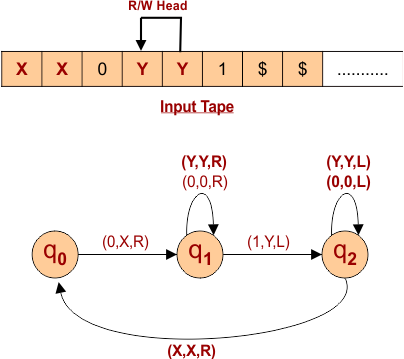Again According To Step 4 and 01, Move Left to Leftmost “0” and update it to “X”, As given below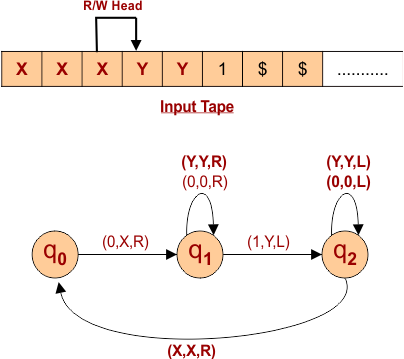Again, According to Step 2 and 3 R/W Head Move to Right To update  first “1” in right side of input tape as explain below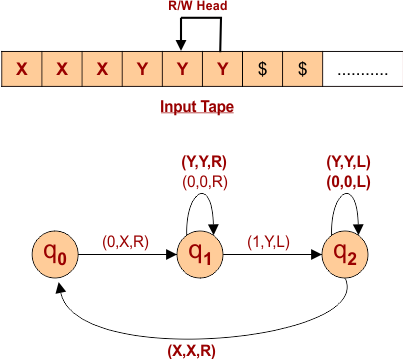At this point,

• all the 0’s and 1’s are updated with X and Y respectively in input tape.
• Current State is q2 and R/W head is moving toward Left of each input “Y”
• As X input occur,  state goes to q0 and R/W Head moves toward Right.
• By consuming all input “Y”, state will be q3.
• As first special symbol “\$” occur, state goes to final state (q4) and R/W head will point the last symbol of considered language.

Hence, language Accepted as given below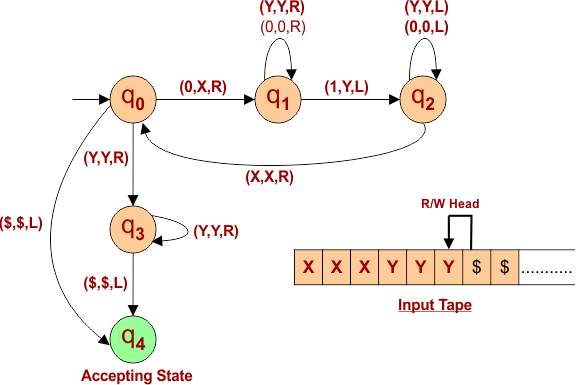Note: For Special symbol (“\$”) at state q0, language is accepted.

Help Other’s By Sharing…